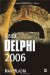# Controlling Loop Execution

Loop execution is normally governed by the condition of the while and repeat-until loops or the start and end values of the for loop. In case we have to exit from the loop earlier, we can use the Break procedure (you can read more about procedures in Chapter 5). The following example shows how to exit from a loop after it executes five times.

Listing 4-11: Breaking out of a loop`program Project1; {\$APPTYPE CONSOLE} uses   SysUtils; var   i: Integer; begin   for i := 1 to 1000 do   begin     WriteLn(i);     if i = 5  then Break;   end;    // for i   ReadLn; end.`### Infinite Loops

As the title suggests, you can write loops that continue to execute forever. In Delphi, only the while and repeat-until loops can be infinite, but in C++ and C# the for loop can also be infinite.

Infinite loops are really easy to write. Since a while loop continues to execute as long as the condition evaluates to True, a simple infinite while loop looks like this:

`while True do   WriteLn('This is an infinite while loop.');`

An infinite repeat-until loop is equally easy to write since it continues to execute as long as the condition evaluates to False:

`repeat   WriteLn('This is an infinite repeat-until loop.'); until False;`
 Note Actually, as you will see in Chapter 23, all Windows applications are based on infinite while loops that are terminated with the Break procedure or other similar procedures.

The following example utilizes an infinite while loop to read user names. To exit from the loop, the user has to enter the word "end."

Listing 4-12: An infinite while loop`program Project1; {\$APPTYPE CONSOLE} uses   SysUtils; var   UserName: string;   UsrCount: Integer; begin   UsrCount := 0;   while True do   begin     Write('Username: ');     ReadLn(UserName);     if UserName = 'end' then     begin       WriteLn('Thank you.');       ReadLn;       Break;     end else     begin       UsrCount := UsrCount + 1;       WriteLn(UsrCount, '. ', UserName);     end;      // if   end;        // while end.`Another way of controlling loop execution is by skipping certain iterations. To skip an iteration, you can use the Continue procedure. The Continue procedure causes the loop to skip to the next iteration without executing the statements that follow it in the block. The following example displays only odd numbers by skipping even iterations.

Listing 4-13: The Continue procedure`program Project1; {\$APPTYPE CONSOLE} uses   SysUtils; var   i: Integer; begin   i := 0;   while i < 20 do   begin     i := i + 1;     { Skip even numbers. }     if i mod 2 = 0  then Continue;     WriteLn('Number ', i, ' is odd.');   end;   ReadLn; end.`The WriteLn statement is only called when the variable i contains an odd number. If the variable i contains an even number, the Continue procedure executes and returns to the first line of the while loop that increments the i variable, thus skipping an iteration.Inside Delphi 2006 (Wordware Delphi Developers Library)
ISBN: 1598220039
EAN: 2147483647
Year: 2004
Pages: 212